TitleCollege Algebra
Answer/Discussion to Practice Problems
Tutorial
45: Exponential EquationsAnswer/Discussion to 1a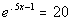This is already done for us in this problem.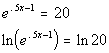*Take the natural log of BOTH sides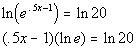*Use the power rule

 Step 4: Solve for x.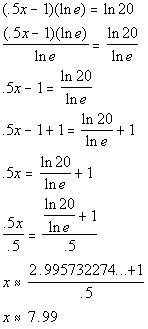*Inverse of mult. by lne is to divide by lne       *Inverse of sub. 1 is add. 1         *Inverse of mult. by .5 is divide by .5   *Use the calculator to find ln 20 *lne is 1Answer/Discussion to 1b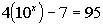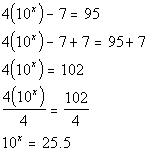*Inverse of sub. 7 is add 7   *Inverse of mult. by 4 is to divide by 4   *Exponential expression isolated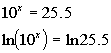*Take the natural log of BOTH sides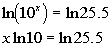*Use the power rule

 Step 4: Solve for x.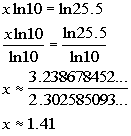*Inverse of mult. by ln 10 is to divide by ln 10   *Use the calculator to find ln 25.5 and ln 10Answer/Discussion to 1cNotice how we have two exponential terms that have different exponents.  We wouldn't be able to isolate both.  We will have to figure out another way to rewrite it so we can continue with the steps.  Note how we have a trinomial and that e to the 2x is e to the x squared.  This means it is quadratic in from.  So we can factor it just like a trinomial of the form.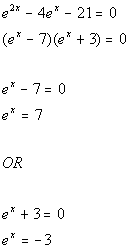*Factor the trinomial of the form.   *Set the 1st factor = 0 *Isolate the exponential expression         *Set the 2nd factor = 0 *Isolate the exponential expression

 Note that since e is a positive base, no matter what the exponent is on x, this exponential expression CANNOT equal -3. So there is only one equation that we can solve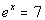.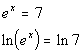*Take the natural log of BOTH sides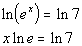*Use the power rule

 Step 4: Solve for x.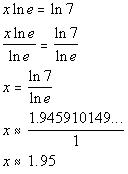*Inverse of mult. by lne is to divide by lne       *Use the calculator to find ln 7 *lne = 1

Last revised on March 23, 2011 by Kim Seward.
All contents copyright (C) 2002 - 2011, WTAMU and Kim Seward. All rights reserved.